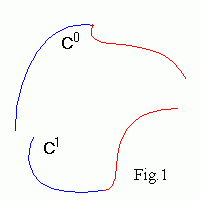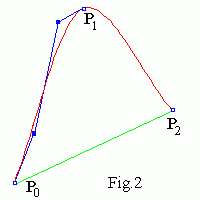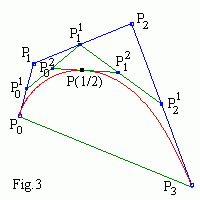# Interpolating Cardinal and Catmull-Rom splines

Continuous curve with a kink in Fig.1 is called C 0 continuous. A curve is C k continuous if all k derivatives of the curve are continuous.Interpolating piecewise Cardinal spline is composed of cubic Bezier splines joined with C1 continuity (see Fig.2). The i-th Bezier segment goes through two neighbouring points Pi , Pi+1 . Derivatives at a point Pi are parallel to the Pi-1Pi+1 line, i.e.
P 'i = (Pi+1 - Pi-1 )/α .
Derivatives at the terminal points P0 , Pn go to the neighbouring nodes. Remember, that a cubic Bezier spline is determined by 4 vectors (e.g. four control points or two endpoints and two derivatives at the points). For the i -th segment we have
B0 = Pi ,   B3 = Pi+1 .
As for Bezier spline
B'(0) = 3(B1 - B0 ) ,   B'(1) = 3(B3 - B2 ) ,
therefore all control points B0,1,2,3 are obtained easy for every segment. Control polygon for the left Bezier segment is shown in Fig.2. Derivatives at P1 are parallel to the P0P2 line. Derivative at the endpoint P0 goes to P1.
One can take different αi values for different points Pi and even different α+,- for the "left" and "right" segments at a point too. For α = 2 we get the Catmul - Rom spline.

Interactive Cardinal spline   Use finger or mouse to move the nearest control point. Use selector to move, add or delete control points. Drag up-down the small blue rectangle to the right to change α.
 move add delete set α

You can compare Cardinal and Bezier spline curves with the same control points.Unfortunately the Cardinal curve cannot be subdivided in two sub-curves which coincide with the initial one. As since Fig.3 shows, that in common case the line P0P3 is not parallel to the P02P12 one and the derivative at P(1/2) is not (P3 - P0 )/α.

Contents   Previous: Interpolating Lagrange curve   Next: Building quadratic B-spline
updated 11 August 2001